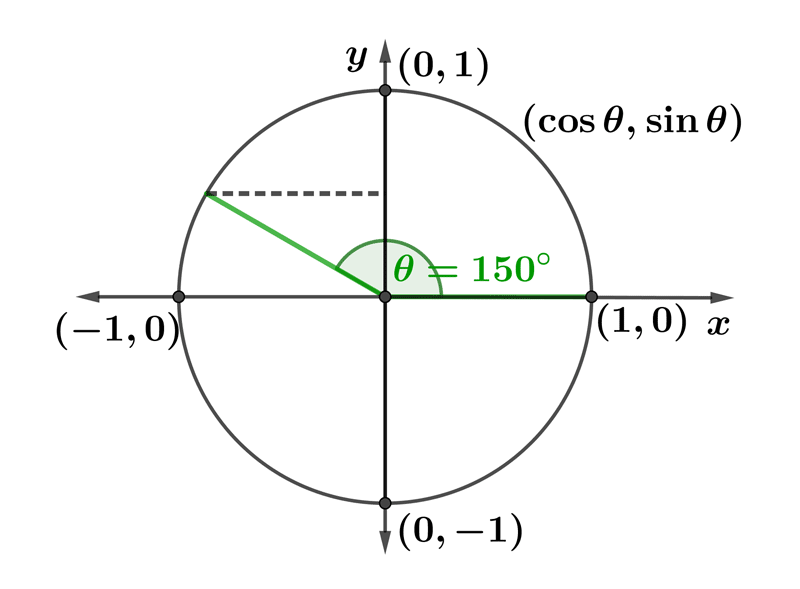Math Calculators, Lessons and Formulas

It is time to solve your math problem

mathportal.org
• Trigonometry
• Trigonometric identities test
• Trigonometric Values of Special Angles

# Trigonometric Values of Special Angles

ans:
syntax error
C
DEL
ANS
±
(
)
÷
×
7
8
9
4
5
6
+
1
2
3
=
0
.
auto next question
calculator
•  Question 1: 1 pts $1^{\circ}=\dfrac{\pi}{360^{\circ}}$ rad
•  Question 2: 1 pts Find the value of $\sin 150^{\circ}.$$\dfrac{1}{2}$ $-\dfrac{\sqrt{3}}{2}$ $-\dfrac{1}{2}$ $\dfrac{\sqrt{3}}{2}$
•  Question 3: 1 pts Find the value of $\tan 30^{\circ}.$
 $\dfrac{\sqrt{2}}{3}$ $\dfrac{\sqrt{3}}{3}$ $\dfrac{\sqrt{3}}{2}$ $\dfrac{\sqrt{2}}{2}$
•  Question 4: 1 pts Find the value of $\cos 120^{\circ}.$
 $-\dfrac{1}{2}$ $\dfrac{1}{2}$ $\frac{\sqrt{3}}{2}$ $-\frac{\sqrt{3}}{2}$
•  Question 5: 2 pts Evaluate.
$\dfrac{1 + \sin (30^{\circ})}{1-\sin 30^{\circ}}=$
•  Question 6: 2 pts Find the correct sign for the given trigonometric values. $$\tan \dfrac{13\pi}{6}$$
 positive negative
•  Question 7: 2 pts Evaluate $3(\cos 30^{\circ})^{2}+2(\sin 30^{\circ})^{2}.$
 $\dfrac{11}{2}$ $\dfrac{5}{4}$ $\dfrac{11}{4}$ $\dfrac{5}{2}$
•  Question 8: 3 pts Evaluate $\cot 300^{\circ} \cdot \cos 300^{\circ} + \tan 300^{\circ} \cdot \sin 300^{\circ}.$
•  Question 9: 3 pts Evaluate $\dfrac{\tan 420^{\circ}}{\cos 60^{\circ}}.$
 $\sqrt{3}$ $2 \sqrt{3}$ $\dfrac{1}{2}$ $1$
•  Question 10: 3 pts If $0^{\circ} <\theta < 360^{\circ}$, then find all possible values of $\theta$ for which $\sin \theta = -\frac{1}{2}$ .
 $30^{\circ}, 210^{\circ}$ $30^{\circ}, 150^{\circ}$ $150^{\circ}, 330^{\circ}$ $210^{\circ}, 330^{\circ}$
•  Question 11: 3 pts If $0^{\circ} < \theta < 360^{\circ}$, then find one possible value of $\theta$ for which $\cot \theta = - \sqrt{3}$ and $\csc \theta = 2.$